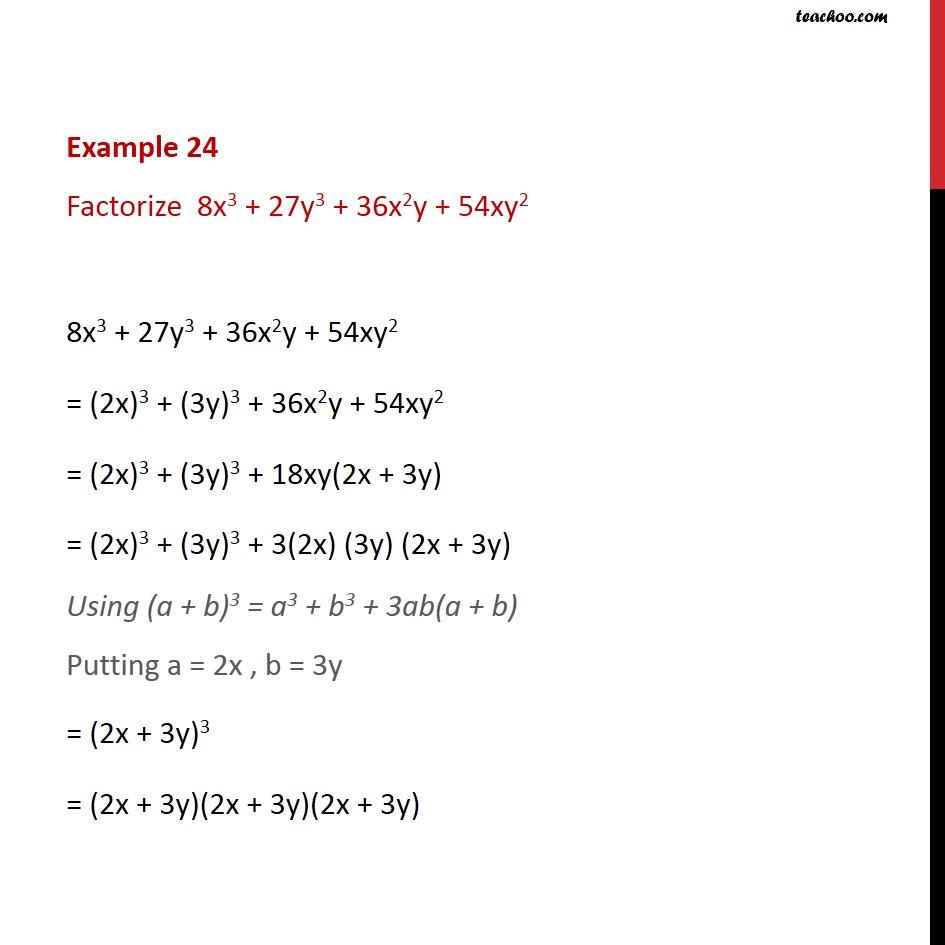Learn all Concepts of Polynomials Class 9 (with VIDEOS). Check - Polynomials Class 91. Chapter 2 Class 9 Polynomials
2. Serial order wise
3. Examples

Transcript

Example 24 Factorize 8x3 + 27y3 + 36x2y + 54xy2 8x3 + 27y3 + 36x2y + 54xy2 = (2x)3 + (3y)3 + 36x2y + 54xy2 = (2x)3 + (3y)3 + 18xy(2x + 3y) = (2x)3 + (3y)3 + 3(2x) (3y) (2x + 3y) Using (a + b)3 = a3 + b3 + 3ab(a + b) Putting a = 2x , b = 3y = (2x + 3y)3 = (2x + 3y)(2x + 3y)(2x + 3y)

Examples

Chapter 2 Class 9 Polynomials
Serial order wise

About the AuthorDavneet Singh
Davneet Singh is a graduate from Indian Institute of Technology, Kanpur. He has been teaching from the past 9 years. He provides courses for Maths and Science at Teachoo.